Function Repository Resource:

# RuledSurfacePlot

Plot a ruled surface

Contributed by: Wolfram Staff (original content by Alfred Gray)
 ResourceFunction["RuledSurfacePlot"][c1,c2,u] plots a ruled surface from the curves c1 and c2 that are parameterized by the variable u. ResourceFunction["RuledSurfacePlot"][c1,c2,{u,umin,umax},{v,vmin,vmax}] plots a ruled surface with u varying from umin to umax and v varying from vmin to vmax.

## Details and Options

A ruled surface is generated by the continuous motion of a straight line passing through two curves c1 and c2, and has a parametrization of the form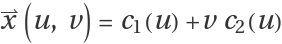, where c1 is the directrix curve and c2 is called the director curve or the ruling.
ResourceFunction["RuledSurfacePlot"] has the same options as ParametricPlot3D.
ResourceFunction["RuledSurfacePlot"][c1,c2,u] is equivalent to ResourceFunction["RuledSurfacePlot"][c1,c2,{u,-π,π},{v,-π,π}].

## Examples

### Basic Examples (2)

A simple ruled surface:

 In:=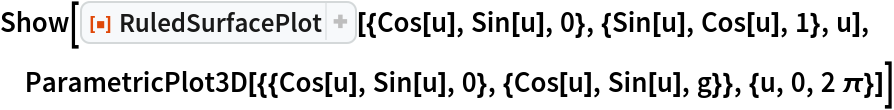Out=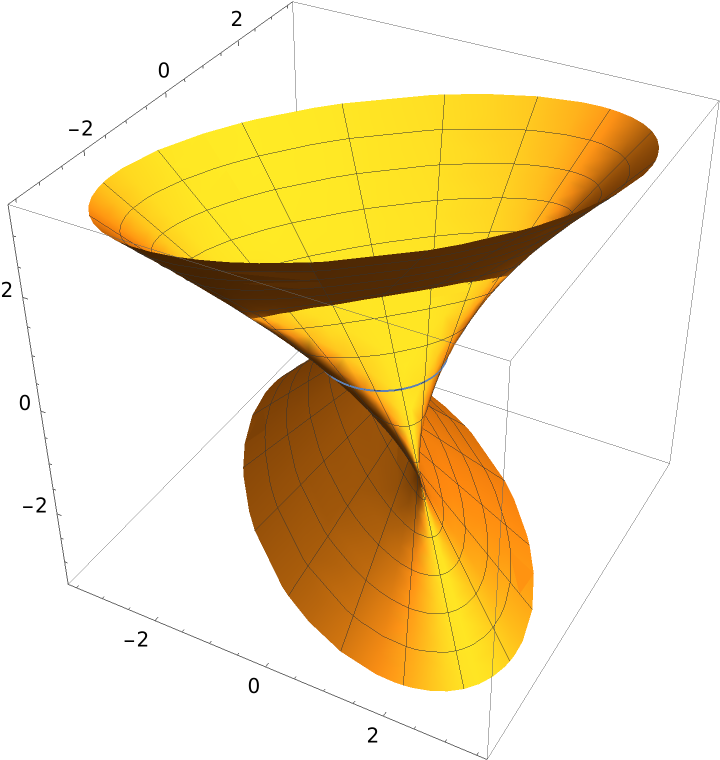This Manipulate shows how the surface is generated, the right line moves around intersecting the two curves, for definite values of u and v:

 In:=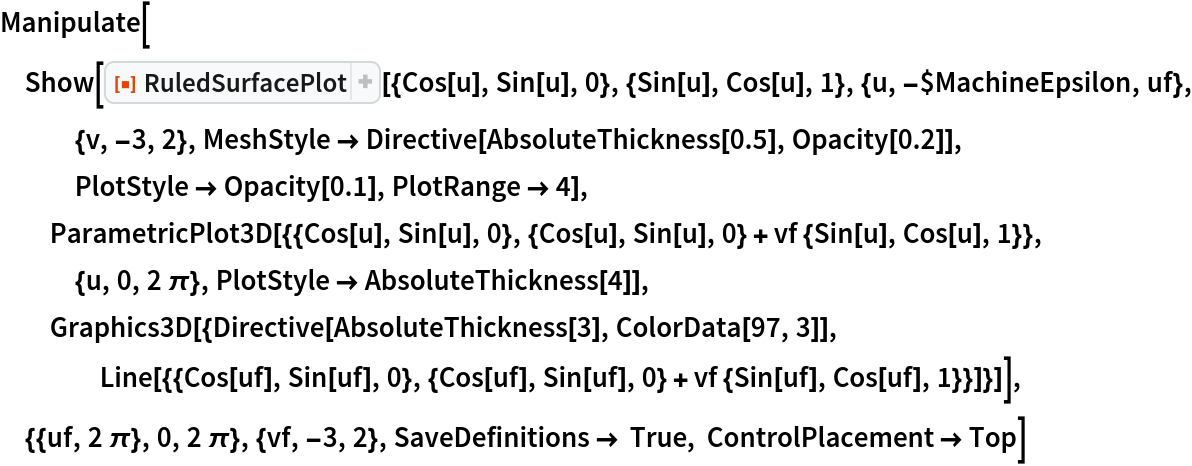Out=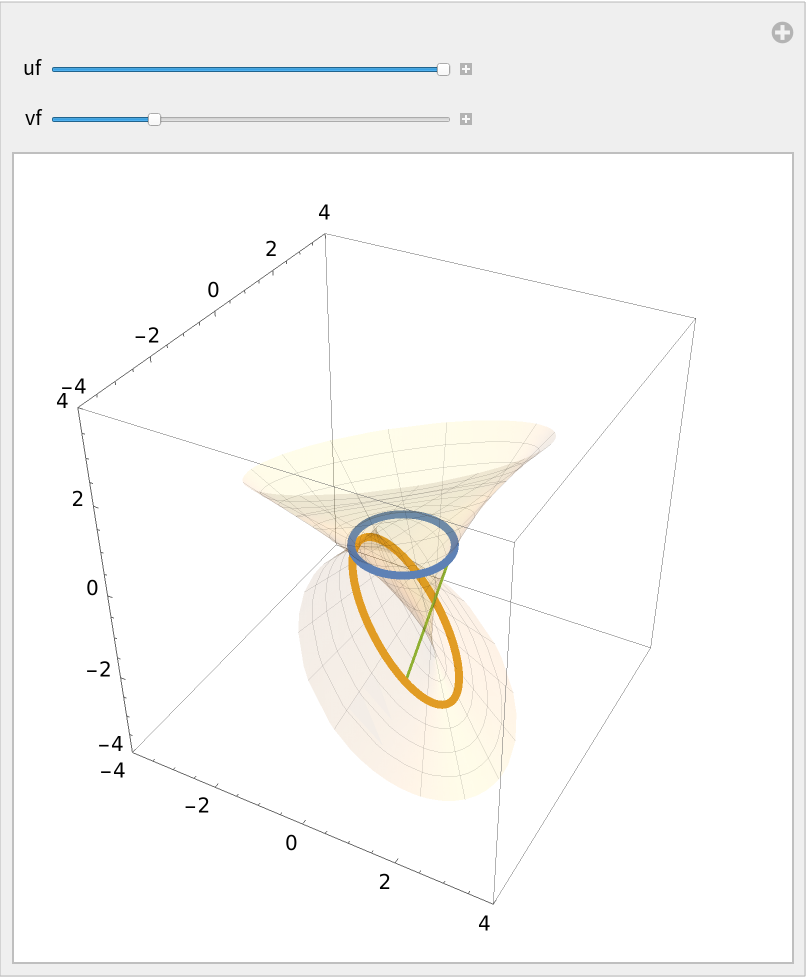### Scope (2)

Modify the default ranges:

 In:=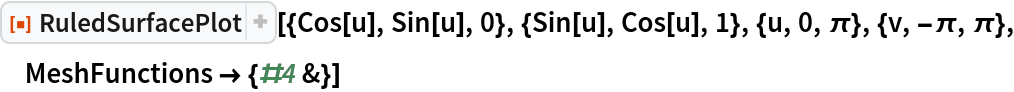Out=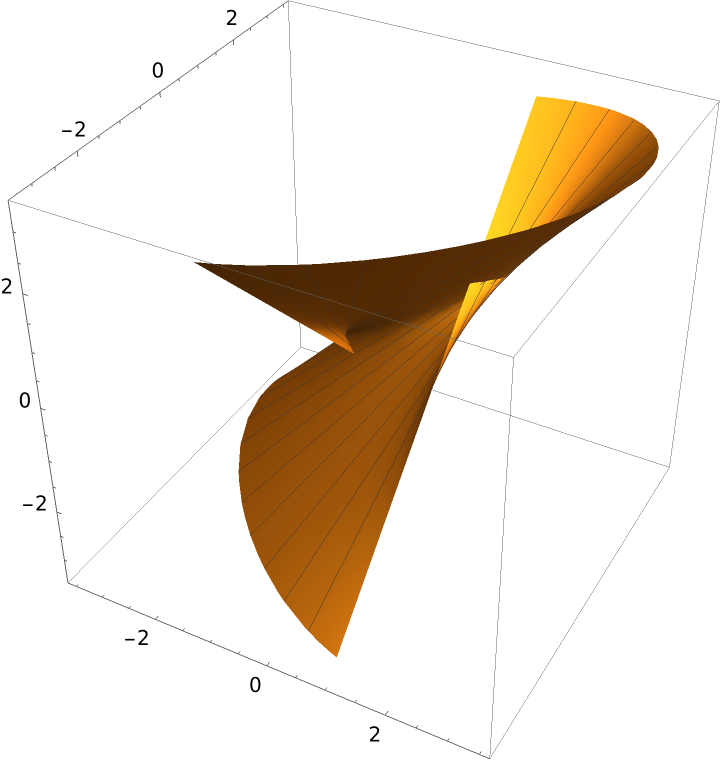Modify the options for enhanced viewing:

 In:=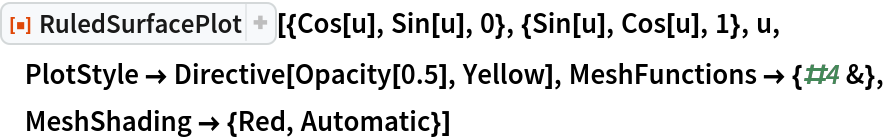Out=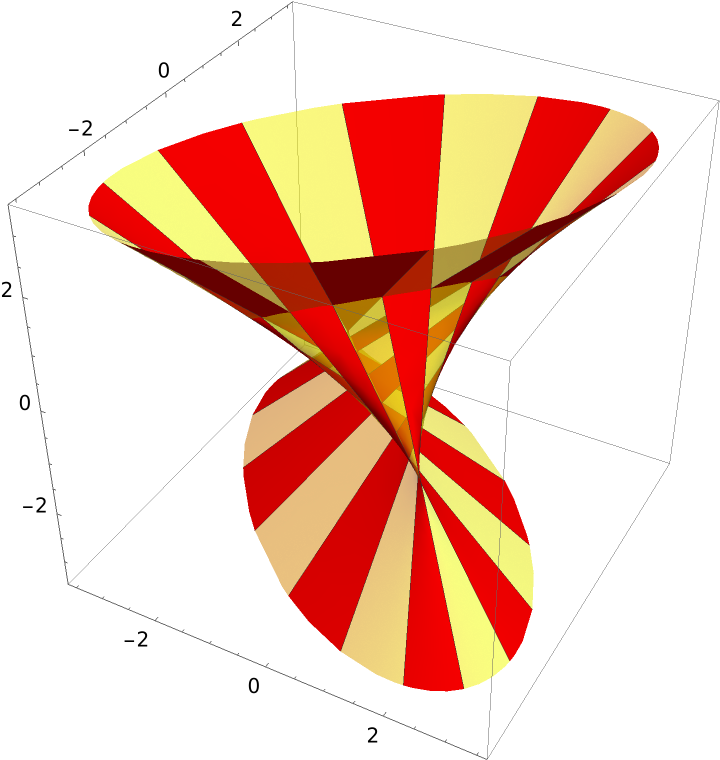### Applications (3)

Plot the generalized hyperbolic paraboloid:

 In:=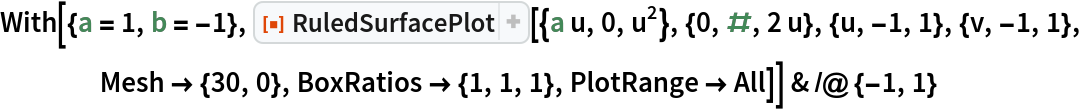Out=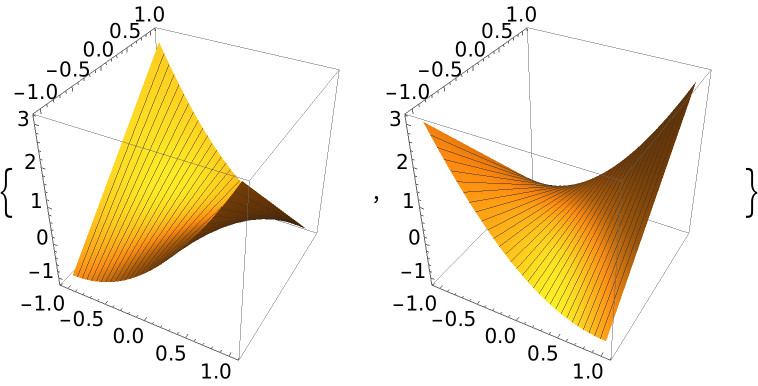Define the Plücker conoid:

 In:=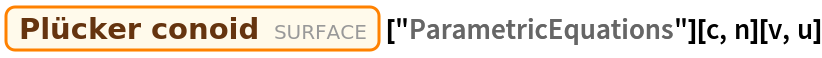Out=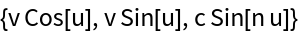Plot the conoid:

 In:=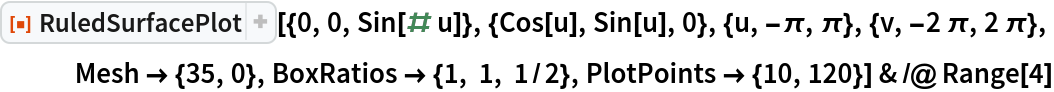Out=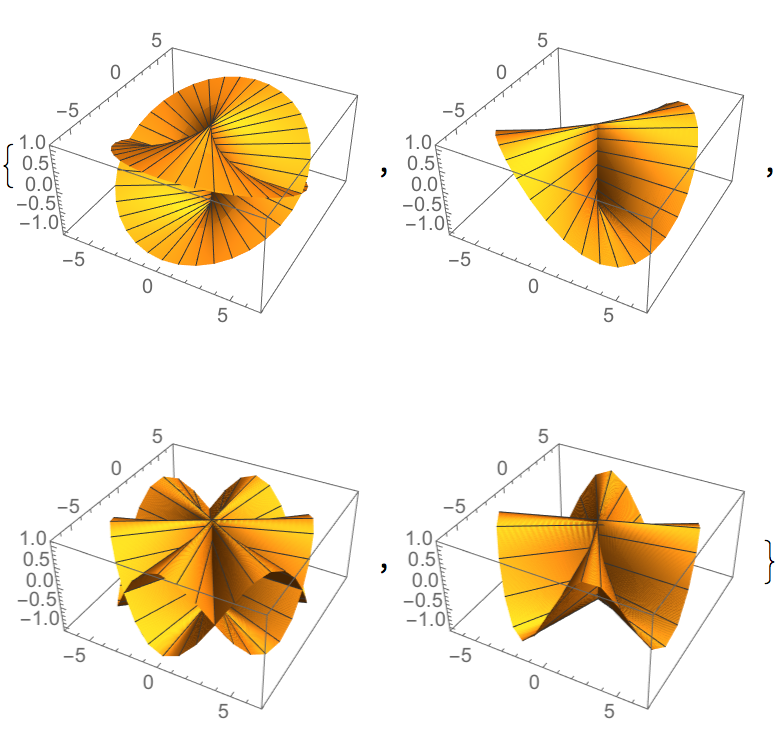The Möbius strip as a ruled surface:

 In:=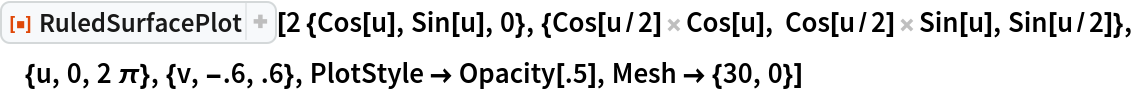Out=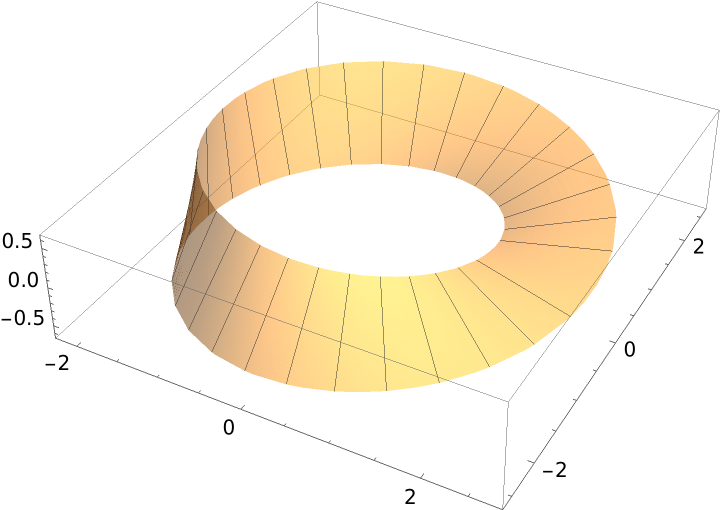A representation of the director curve c2(u):

 In:=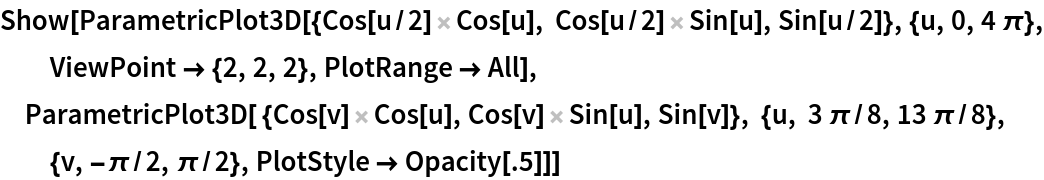Out=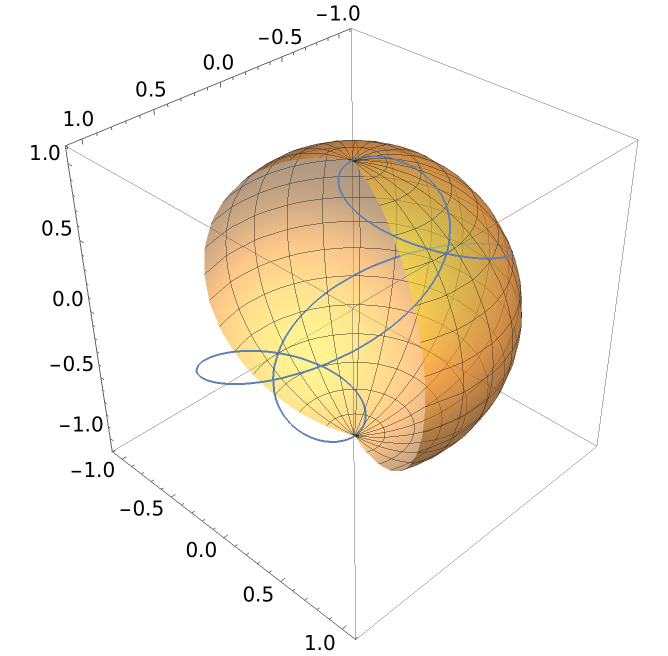### Properties and Relations (4)

An elliptical hyperboloid is doubly ruled because it can be parametrized in two ways:

 In:=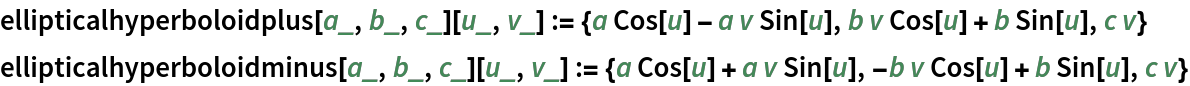The "minus" chart could easily have been defined in terms of the "plus" one:

 In:=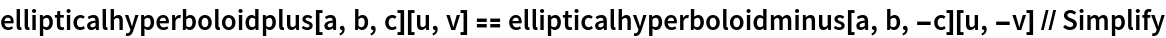Out=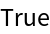Plot both cases:

 In:=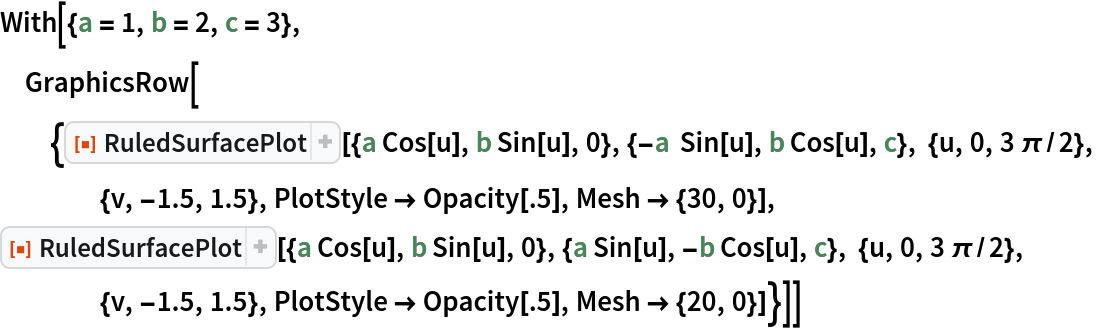Out=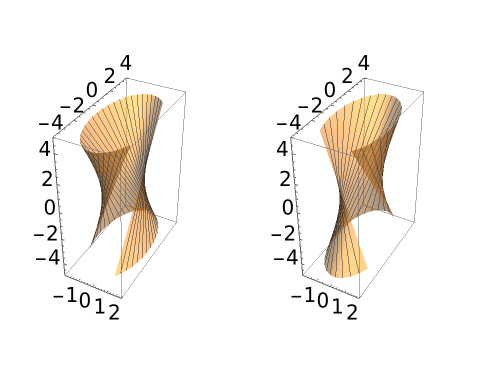The Gaussian curvature of a ruled surface is everywhere nonpositive:

 In:=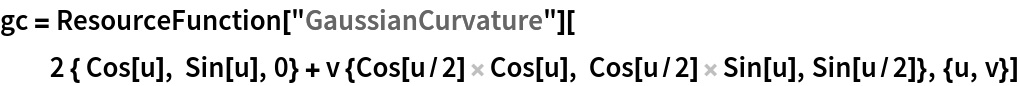Out=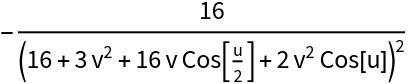In:=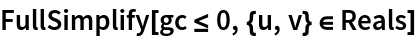Out=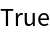This plot exhibits its minima, corresponding to regions which are especially distorted:

 In:=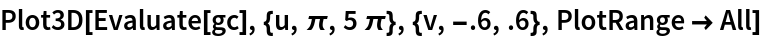Out=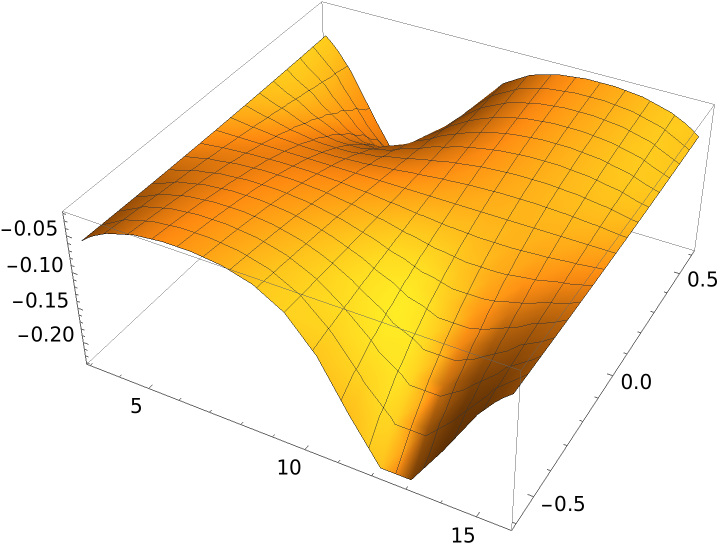Generalized cylinders and cones have the form of a ruled surface:

 In:=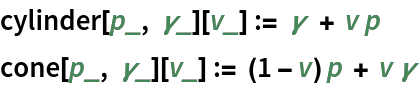A figure-eight curve:

 In:=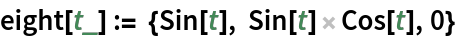Parametrizations of a generalized cylinder and cone using a figure-eight curve:

 In:=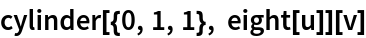Out=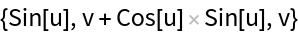In:=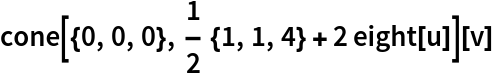Out=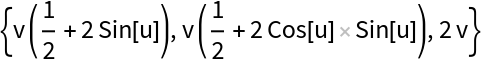Plot the surfaces:

 In:=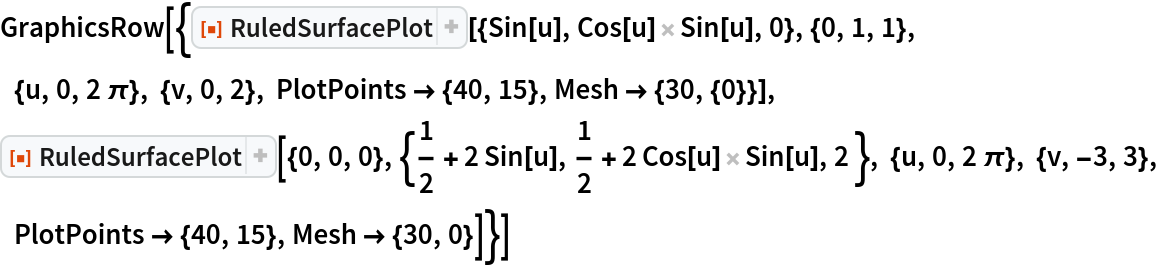Out=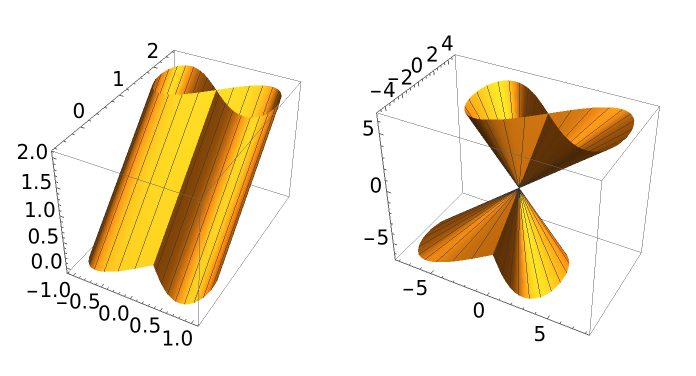If the Gaussian curvature of a ruled surface is everywhere zero, then it is said to be a flat surface:

 In:=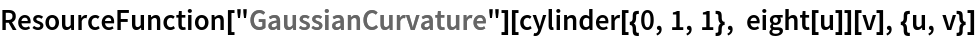Out=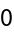In:=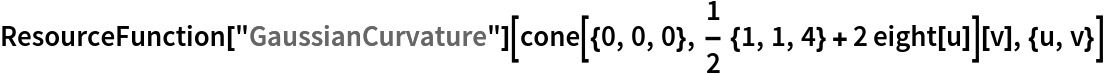Out=The tangent developable of a space curve α is a ruled surface, whose director curve is the unit tangent vector field to α:

 In:=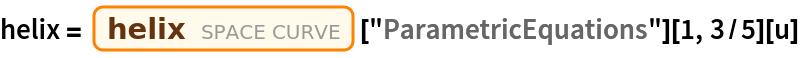Out=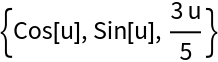In:=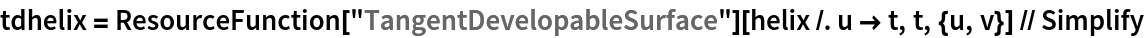Out=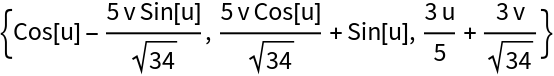In:=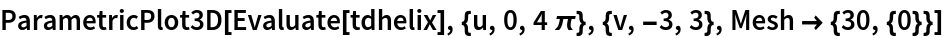Out=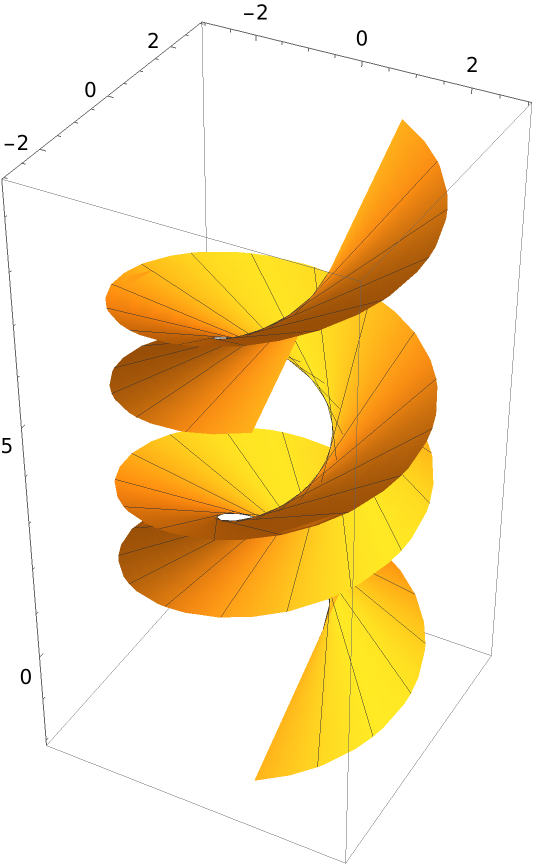In:=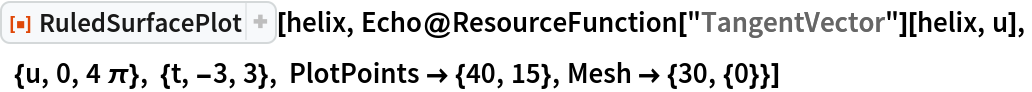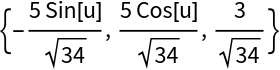Out=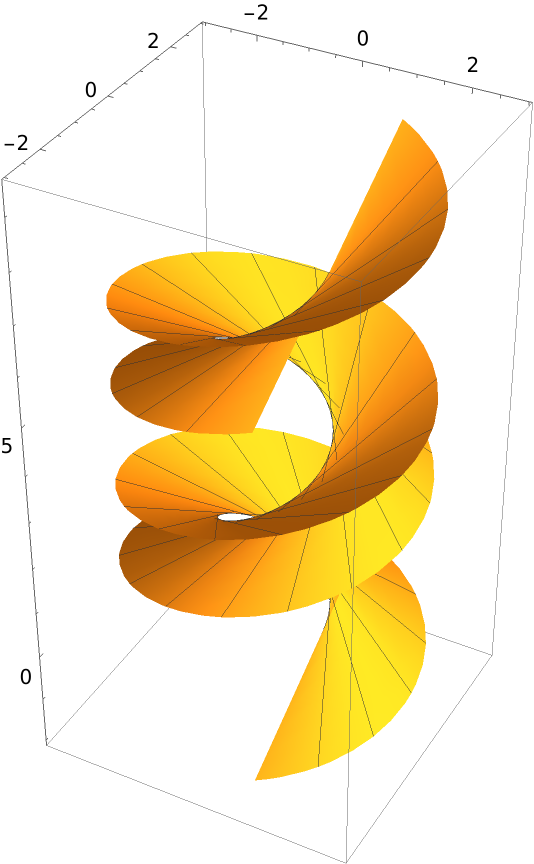Enrique Zeleny

## Version History

• 1.0.0 – 26 July 2021